Next: 2.2 Operation Principle Up: 2. The Photolithography Process Previous: 2. The Photolithography Process

# 2.1 Some Fundamental Considerations

The performance of optical lithography, its limitation and possible strategies for further enhancements can be best explained by considering the following two figures of merit. The resolution W, i.e., the minimal printable feature size, is given by the expression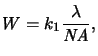(2.1)

whereas the depth of focus  DOF, i.e., the range over which the image is adequately sharp, is governed by the relation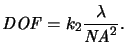(2.2)

In the above equations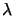and NA are the actinic wavelength and the numerical aperturea of the exposure tool, respectively. These two quantities will be discussed in detail throughout the subsequent sections. k1 and k2 are characteristic constants of the specific lithography process. They are both in the range of 0.5 to 1.0. Under special conditions they can be theoretically calculated. The Rayleigh limit'' for example describes the resolving power of microscope objectives and gives k1 = 0.61 and k2 = 1.0 [11, p. 415]. In practical semiconductor lithography, k1 and k2 generally depend on a large number of tool, resist, and process parameters, the type of the mask and the pattern being imaged, as well as the requirements of the shape and allowed size range of the developed resist profile.

From (2.1) follows that the resolution can be improved in three ways: by shortening the exposure wavelength, by increasing the numerical aperture NA, and by decreasing the value of k1. As shown in Figure 2.1, all three strategies were pursued simultaneously in the past, and are projected to continue for the foreseeable future.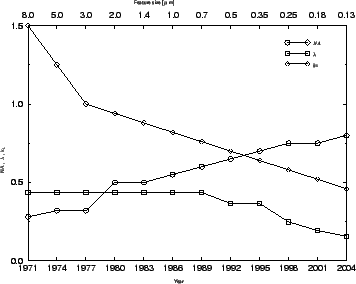At the same time as the resolution is increased by decreasing the wavelength and increasing the numerical aperture the depth of focus is reduced (cf. (2.2)). The reduction in depth of focus has become a formidable problem and has led to the development of planarization techniques for resists like top-surface imaging or multilayer systems. Because of the inverse square dependency on the numerical aperture, the depth of focus becomes extremely shallow for high- NA tools. The dependence on the wavelength is less severe due to the linear relation. Eliminating NA between (2.1) and (2.2) yields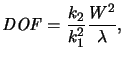(2.3)

which explicitly shows that for the same process parameters k1 and k2 and the same resolution W a shorter wavelengthgives a larger depth of focus. From the viewpoint of the resolution, this is the motivation for exploring shorter wavelengths, even when a longer wavelength seems to be adequate. Another observation is that a smaller k1 increases the depth of focus quadratically, which has motivated various resolution enhancement techniques like advanced illumination schemes, phase-shifting masks, and in-lens filtering. The fundamental relations are illustrated in Figure 2.2.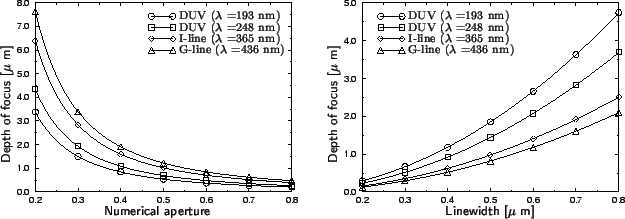#### Footnotes

... aperturea
The numerical aperture  NA of a lens is the sine of its half acceptance angle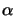, i.e., NA = sin(/2). An exact definition is given in the context of aerial image simulation in Chapter 4 on page.Next: 2.2 Operation Principle Up: 2. The Photolithography Process Previous: 2. The Photolithography Process
Heinrich Kirchauer, Institute for Microelectronics, TU Vienna
1998-04-17